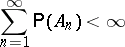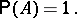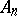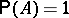Difference between revisions of "Borel-Cantelli lemma"

A frequently used statement on infinite sequences of random events. Let $A_1,\dots, A_n, \dots$ be a sequence of events from a certain probability space and let $A$ be the event consisting in the occurance of (only) a finite number out of the events $A_n$, $n=1,2\dots$. Then, according to the Borel–Cantelli lemma, if(*)If the eventsare mutually independent, thenor 0, depending on whether the seriesconverges or diverges, i.e. in this case the condition (*) is necessary and sufficient for; this is the so-called Borel criterion for "zero or one" (cf. Zero-one law). This last criterion can be generalized to include certain classes of dependent events. The Borel–Cantelli lemma is used, for example, to prove the strong law of large numbers.